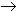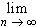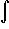#Interactive Real Analysis

Next | Previous | Glossary | Map

## 8. Sequences of Functions

### 8.1. Pointwise Convergence

Previously we discussed numeric sequences and series; now we are interested in investigating the convergence properties of sequences (and series) of functions. In particular, we would like to know:

1. How do we define convergence if we have a sequence of functions instead of a numeric sequence?
2. Is the limit of a convergent sequence of functions again a function?
3. If we have a sequence of functions, each of which is continuous, is the limit function again continuous?
4. If we have a sequence of functions, each of which is differentiable, is the limit function again differentiable?
5. If we have a sequence of functions, each of which is integrable, is the limit function again integrable?

It turns out that sequences and in particular series of functions can be quite complex but at the same time they can give rise to functions with interesting and new properties. But - first things first: we need to talk about how to "group" functions into (happy?) families.

 Definition 8.1.1: Function Family A family of functions is defined as a function of two variables f: D x SR, DR where the second variable is called parameter. A family of functions is usually written as { fc(x) }, where the variable xD and the parameter cS. The set D is called the domain of the function family, while S is the parameter set for the family.

At first this definition might look confusing, especially since it talks about functions of two variables. The trick, though, is to consider only the first variable as a true variable, while the second one is considered a parameter that's kept constant as x varies. In other words, for each fixed parameter c we get a regular, good old function of x. Perhaps an example helps:

 Example 8.1.2:Consider the family of functions { fc(x) } = { x2 - c }. What is the (natural) domain of the family? What is the (natural) parameter set of the family? List three particular members of the family. How many members does this family have?Consider { fn(x) } = { n / (x - n) }, where n is a natural number. What is the domain of this family? What is the parameter set?

The two families above are fundamentally different: the first has uncountably many members while the second one consists of only countably many functions indexed by the numbers 1, 2, 3, .... Thus, the second one should be easier and families of functions where the parameters are natural numbers get their own name:

 Definition 8.1.3: Sequence of Function A family of functions where the parameter set is the set of natural numbers is called a sequence of functions. We write it as { fn(x) }.

In other words, if we have a sequence of functions such as, for example, { fn(x) } = { xn } then:

• For each fixed n we get a new function. For example if n = 2 we have the standard square function f(x) = x2.
• If we fix an x, on the other hand, we get a numeric sequence. For example if x = 2 we get the familiar geometric sequence fn(x) = { 2n }.

Here is another, perhaps more interesting example of a function sequence:

 Example 8.1.4:Define { fn(x) }, where fn(x) = max(n - n2 |x - 1/n|, 0) and x[ 0, 1 ]. Describe the elements of this function sequence.

We have seen that for each fixed x a function sequence turns into a (simple) numeric sequence. In the picture below, for example, we have drawn several members of a particular function sequence:If we fix a value for x, say x = 0.2, we get a numeric sequence. In the picture above you can see that

x2 = f2(0.2) = 0.8, x3 = f3(0.2) = 1.8, x4 = f4(0.2) = 3.2, and x5 = f5(0.2) = 5.0

At first glance looks like xn = fn(0.2) is a sequence diverging to infinity, but looking at the pattern for the functions fn we guess that xn must eventually be zero for n > N. Thus the sequence { xn } should converge to zero (this is of course the sequence from the above example).

We can use the fact that function sequences reduce to numeric sequences for fixed values of x to define our first type of convergence:

 Definition 8.1.5: Pointwise Convergence A sequence of functions { fn(x) } with domain D converges pointwise if for each fixed x0D in the domain the numeric sequence { fn(x0) } converges. In other words: for each fixed x0 and any given> 0 there exists a positive integer N such that | fn(x0) - L | <whenever nN for some limit L. Note that the limit L depends on x0, while the integer N depends on x0 and.

Since the limit L in the definition depends on x, a pointwise converging sequence of function defines a new function.

 Proposition 8.1.6: Pointwise Convergence defines Function If { fn(x) } converges pointwise, thenfn(x) = f(x) is a well-defined function.

So we answered the first two questions posed at the beginning of our section: we have defined a type of convergence for function sequences such that if they converge they converge to a new function.

 Example 8.1.7Let fn(x) = max(n - n2 |x - 1/n|, 0), x[ 0, 1 ]. Show that this sequence converges pointwise to the function f(x) = 0 for x[ 0, 1 ].Show that fn(x) = xn, x[ 0, 1 ] converges pointwise and identify the limit function.

So much for the good news. It turns out that pointwise convergence is not enough to preserve properties of functions. In other words, if a sequence of functions has property X and converges pointwise, then the limit function may or may not have property X.

 Example 8.1.8Find a pointwise convergent sequence of functions, each of which is continuous, but whose limit function is not continuous.Find a pointwise convergent sequence of differentiable functions such that the limit function is continuous but not differentiable.Find a pointwise convergent sequence of Riemann-integrable functions whose limit is not Riemann-integrable.Find a pointwise convergent sequence of differentiable functions whose limit is differentiable but the sequence of derivatives does not converge.Find a pointwise convergent sequence of Riemann-integrable functions whose limit is Riemann-integrable butfn(x) dx #fn(x) dx =f(x) dx

This is clearly bad news. We would like a type of convergence that at least preserves some properties of functions. Clearly pointwise convergence does not, so that we will have to define another, more stringent type of convergence - in our next chapter.

Next | Previous | Glossary | Map